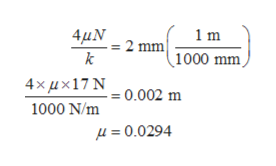Question
1 views

In the system shown,m= 2 kg, k= 1000 N/m, theta= 30 degrees and the friction coefficient between the block and the surface is u.

(a) If the block is displaced from equilibrium and released, the decrease in amplitude per cycle is 2 mm. Determine the coefficient of friction u.

(b) If the initial displacement is 5 cm from equilibrium, determine the time and position when the block comes to rest.

check_circle

Step 1

Draw the free body diagram.

Step 2

a)

Calculate the normal reaction.

Step 3

Now, the decrease in ampli...help_outlineImage Transcriptionclose4μΝ 1m 2 mm 1000 mm 4xμx17 , =0.002 m 1000 N/m μ= 0.0294 fullscreen

### Want to see the full answer?

See Solution

#### Want to see this answer and more?

Solutions are written by subject experts who are available 24/7. Questions are typically answered within 1 hour.*

See Solution
*Response times may vary by subject and question.
Tagged in

### Mechanical Engineering